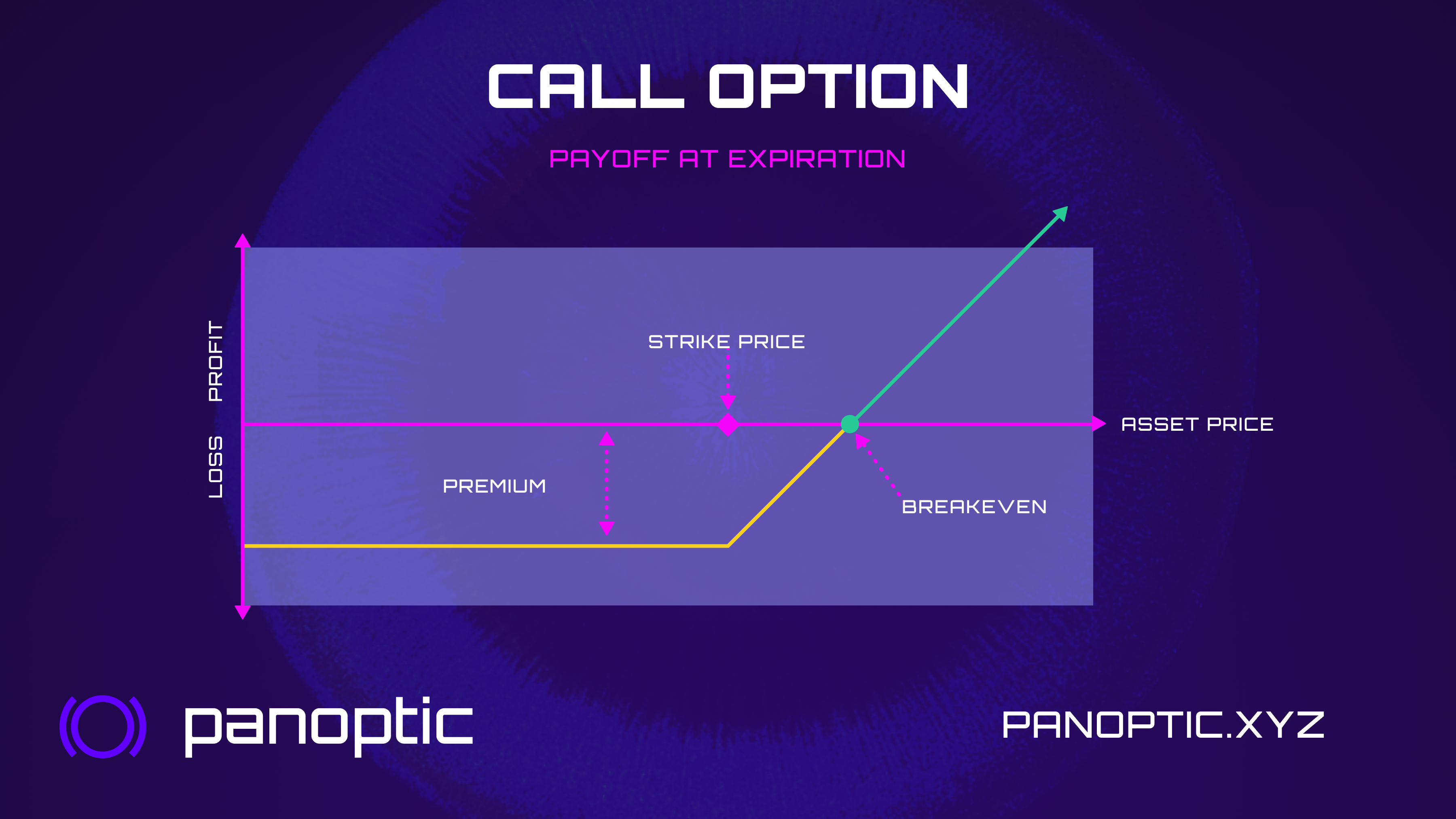# Options Basics

What options are and how to manage them.

## What are Options?​

Options are an agreement between two parties for the right, but not the obligation, to buy (or sell) an asset for a fixed price at a pre-determined time. Hence, each option has a

• Strike: the agreed-upon price the asset can be bought/sold for
• Expiry: a time in the future when the transaction has to occur
• Premium: the value of the option, or how much the buyer pays for the right to buy/sell the assetOptions provide traders with a versatile tool for managing risk and generating profits.

• By buying or selling options, traders can speculate in a capital efficient manner on the price movement of a security. This is done by taking a long or short position without having to own the underlying asset.
• Options can also be used to manage portfolio risk, by hedging against potential losses in other investments.
• In addition, options can be used to generate income, through the writing of options contracts.

Because options allow traders to take on different levels of risk, and also defined-risk positions, they are a useful tool for managing financial portfolios.

## How are Options Typically Priced?​

The value of an option called the premium used to be difficult to determine.

But following the development of Nobel-prize winning mathematical models by Black, Scholes, and Merton, an optimal price could be derived in a way that buyers and sellers both agree on the option contract's price.

So, how does it work? In the simplest case (i.e. for European options) the Black-Scholes Model (BSM) considers the following variables:

• Spot price ($S$): The current price of the underlying asset.
• Strike price ($K$): The predetermined price at which the option can be exercised.
• Time to expiration ($T$): The time remaining until the option expires.
• Volatility ($\sigma$): The annualized standard deviation of the underlying asset's returns, reflecting fluctuations in the underlying asset's price.
• Risk-free interest rate ($r$): The interest rate on a risk-free investment, typically a government bond.

Using these variables, the Black-Scholes equation calculates the fair value of an option. The equation is different for call and put options, but they share the same core components, namely:

$N(d_1)$ and $N(d_2)$: These are probability values derived from the cumulative standard normal distribution. They represent the likelihood of the option being exercised. More precisely,

• $d_1$: can be thought of as a measure of how much the option is "in the money" relative to its risk, incorporating factors such as the stock price, strike price, time to expiration, volatility, and risk-free interest rate. A higher d1 value indicates that the option is more likely to be exercised, as the stock price is expected to be further away from the strike price. This increased likelihood of exercise is reflected in a higher N(d1) value. The formula for $d_1$ is: $d_1 =\frac{(\ln\left(\frac{S}{K}\right) + (r + \frac{\sigma^2}{2}) \cdot T)}{\sigma \cdot \sqrt{T}}$

• $d_2$ can be understood as a measure of the expected payoff when the option is exercised, considering the time value of money. A higher $d_2$ value indicates that the option's exercise price is more favorable compared to the current stock price and risk-free interest rate, which is reflected in a higher N(d2) value. $d_2$ is derived from $d_1$ as follows: $d_2 = d_1 - \sigma \cdot \sqrt{T}$

Present value factor ($e^{-rT}$): This factor discounts the value of the option based on the risk-free interest rate and time to expiration.

Given the discussion above, the Black-Scholes equation for call options is as follows:

• Call option value = $S \cdot N(d_1) - K e^{-rT} \cdot N(d_2)$

For put options, the equation is:

• Put option value = $K \cdot e^{-rT} \cdot N(-d_2) - S \cdot N(-d_1)$

It is worth mentioning that, while the BSM is widely used, it has some limitations:

• Assumes constant volatility: The equation assumes that the underlying asset's volatility remains constant, which is not always the case in reality.
• Ignores dividends: The original model doesn't account for dividends paid by the underlying asset, which can affect the option's value.
• Assumes European-style options: The model is based on European-style options, which can only be exercised on the expiration date. It may not be accurate for American-style options, which can be exercised any time before expiration.
• Assumes a constant risk-free rate: The equation assumes that the risk-free rate remains constant, which is not always the case in reality.
• Assumes GBM: The model assumes the underlying asset's price follows a geometric Brownian motion, which is not always the case in reality (especially for stablecoins!).

## Is Panoptic the same?​

Not quite. Panoptic presents a new type of option: an oracle-free, perpetual option. By this we mean that:

• Panoptions do not have an expiration date
• The option premia is not based on pricing by market makers (who use BSM), but rather on trading activity in the underlying asset's spot market.

We will discuss these concepts in the following sections.